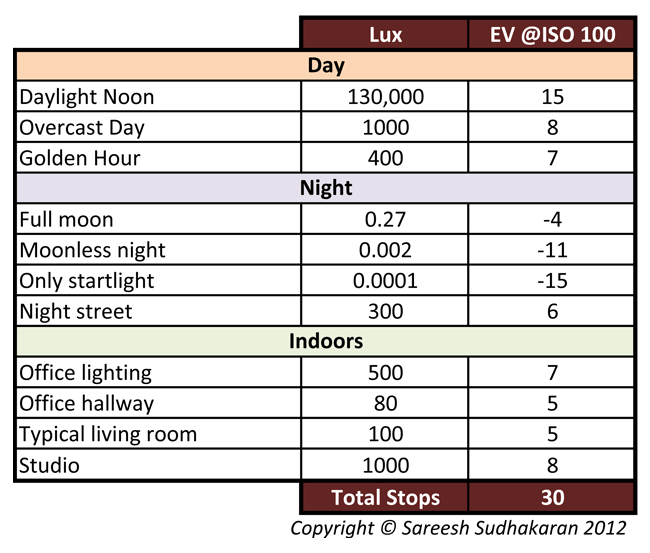Categories

# Notes by Dr. Optoglass: How to Read Light for Exposure

Topics Covered:

• Light meter – Incident and Reflective
• Lux, EV and Common Scenarios
• The Inverse Square Law

Art is never finished, only abandoned – Leonardo da Vinci

Now that we know what an exposure is, we are ready to measure it. There are two ways in which to do this:

• Using an incident light meter
• Using a reflected light meter

Light meterA light meter reads light and presents this information to you in usable ways – namely as aperture, shutter, ISO, lux, etc. Every digital camera has an in-built light meter, but some professionals don’t completely rely on them. No matter which meter you use, the important thing to remember is that the light meter must be calibrated – in other words, it must be accurate.

Light meters tell you directly what settings you can use to get a good exposure, and from that point on the ball is in your court.

Reflected light meter

The light meters in most cameras are reflected light meters. What this means is that the light meter is designed to read light that is reflected off objects. The meter is not pointed to the source of the light, but to the scene instead. Light hits the objects in the scene and is reflected. This reflected light is measured by the meter to give the right exposure value.

The formula for Reflected exposure is derived as:Where
N is the f-number
t is the shutter speed in seconds
L is the scene luminance in cd/m2 or nits
S is the ISO arithmetic speed
K is the reflected-light meter calibration constant

Incident light meter

An incident light meter is designed to read light from the source itself. You use this meter where the object will be in the scene to read how the light falls on it.

The formula for calculating exposure for incident light is derived as:Where
E is the illuminance in lux
C is the incident-light meter calibration constant

Which is better – reflected or incident? Neither. They are different tools for different jobs. If you are presented with a scene over which you have no lighting control, a reflected meter will be fine. However, if you are in a studio setting and need to know precisely what each light is doing, then an incident light meter is valuable.

What you might have noticed from the above formulas is that EV is totally dependent on the meter calibration constant. Each meter has a different value. A typical value for K might be 12.5 (Canon and Nikon), while a typical value of C for a hemispherical sensor meter might be around 320 (Minolta) to 340 (Sekonic).

For a particular meter-camera combination, once you have the values for the constants, and you know your ISO setting, there is a direct relationship between Luminance (nits), Illuminance (lus) and EV. Luckily, the meters tell you exactly which f-number and shutter speed to use at any given ISO – so you don’t have to worry about making any calculations.

Here are a few rule of thumb values of EV and lux for common lighting scenarios:Inverse Square LawLight intensity is inversely proportional to the square of the distance from the source. Doubling the distance reduces illumination to one quarter.

E.g. if my light reading is 100 lux at 2m, then if I move away to 4m the illuminance will be 25 lux. If I move closer to 1m, then the illuminance will be 400 lux. This is the fundamental reason why f-numbers are related to ?2.

Now you are ready to know what the f-number of the eye is.

Takeaways:

• A light meter measures light and returns values that make practical work easy.
• A reflected light meter reads reflected light, while an incident light meter reads direct light.
• There are about 30 stops of light in the real world.
• Light intensity is inversely proportional to the square of the distance from the source.